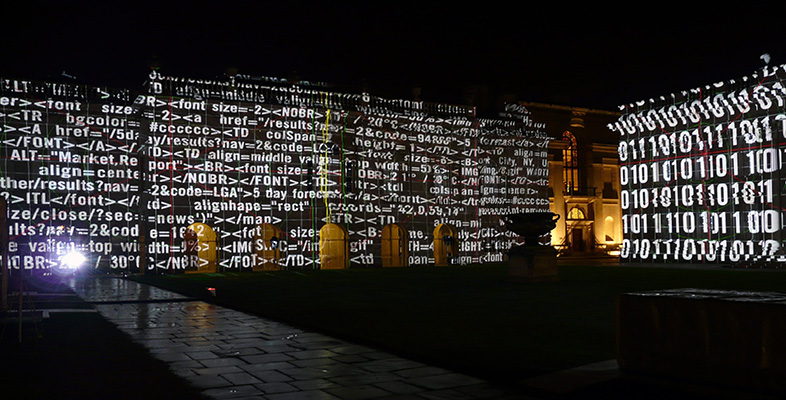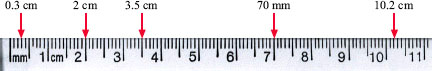Using numbers and handling data

Start this free course now. Just create an account and sign in. Enrol and complete the course for a free statement of participation or digital badge if available.

Free course

# 1.3 Marking decimals on a scale

Figure 2 shows a picture of a ruler. The major units are marked in centimetres (1 to 11 cm), whilst the intervals between the centimetres have each been split into ten equal, smaller units. These minor units are therefore tenths of a centimetre, commonly known as 'millimetres'. (There are 10 millimetres in 1 centimetre.) A similar type of decimal scale is used on many devices that you will work with, such as syringes, gas pressure gauges and pH meters, even though the physical quantities they measure, and therefore their units of measurement, are different.

In Figure 2 several numbers have been highlighted to allow you to relate the way a decimal number is written to the quantity that number represents. For instance, if our units of measurement are centimetres, then we can see that 0.3 cm is less than 1 cm (it is actually 3 millimetres). Similarly, 2 cm is less than 3.5 cm. However, 70 mm is less than 10.2 cm, because 70 mm means the same as 7 cm.Figure 2 Units and tenths of units represented on a ruler

Now practise placing decimal values on a similar scale for yourself with practice questions 4 to 8.

Right click and open the practice questions [Tip: hold Ctrl and click a link to open it in a new tab. (Hide tip)] in a separate window, then you can switch easily between the course text and the questions.

S110_1